Related Articles
Time Series Plot or Line plot with Pandas
• Last Updated : 26 Nov, 2020

Prerequisite: Create a Pandas DataFrame from Lists

Pandas is an open-source library used for data manipulation and analysis in Python. It is a fast and powerful tool that offers data structures and operations to manipulate numerical tables and time series. Examples of these data manipulation operations include merging, reshaping, selecting, data cleaning, and data wrangling. This library allows importing data from various file formats like SQL, JSON, Microsoft Excel, and comma-separated values. This article explains how to use the pandas library to generate a time series plot, or a line plot, for a given set of data.

A line plot is a graphical display that visually represents the correlation between certain variables or changes in data over time using several points, usually ordered in their x-axis value, that are connected by straight line segments. The independent variable is represented in the x-axis while the y-axis represents the data that is changing depending on the x-axis variable, aka the dependent variable.

To generate a line plot with pandas, we typically create a DataFrame* with the dataset to be plotted. Then, the plot.line() method is called on the DataFrame.

Syntax:

DataFrame.plot.line(x, y)

The table below explains the main parameters of the method:

Additional parameters include color (specifies the color of the line), title (specifies the title of the plot), and kind (specifies which type of plot to use). The default variable for the “kind” parameter of this method is ‘line’. Therefore, you don’t have to set it in order to create a line plot.

Example 1:

The example illustrates how to generate basic a line plot of a DataFrame with one y-axis variable. Use pandas in Python3 to plot the following data of someone’s calorie intake throughout one week, here is our dataframe.

Code:

## Python3

 `import` `pandas as pd`` ` `# Create a list of data to be represented in x-axis``days ``=` `[ ``'Saturday'` `, ``'Sunday'` `, ``'Monday'` `, ``'Tuesday'` `,``        ``'Wednesday'` `, ``'Thursday'` `, ``'Friday'` `]`` ` `# Create a list of data to be ``# represented in y-axis``calories ``=` `[ ``1670` `, ``2011` `, ``1853` `, ``2557` `,``            ``1390` `, ``2118` `, ``2063` `]`` ` `# Create a dataframe using the two lists``df_days_calories ``=` `pd.DataFrame(``    ``{ ``'day'` `: days , ``'calories'` `: calories })`` ` `df_days_calories`

Output: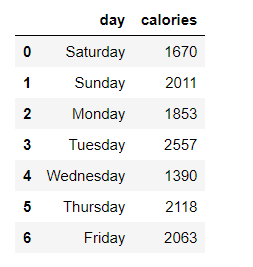Now, Plotting the variable.

## Python3

 `# use plot() method on the dataframe``df_days_calories.plot( ``'day'` `, ``'calories'` `)`` ` `# Alternatively, you can use .set_index ``# to set the data of each axis as follows:``# df_days_calories.set_index('day')['calories'].plot();`

Output: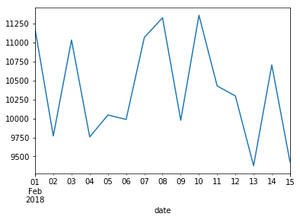Example 2:

This example explains how to create a line plot with two variables in the y-axis.
A student was asked to rate his stress level on midterms week for each school subject on a scale from 1-10 (10 being the highest). He was also asked about his grade on each midterm (out of 20).

Code:

## Python3

 `import` `pandas as pd`` ` `# Create a list of data to``# be represented in x-axis``subjects ``=` `[ ``'Math'` `, ``'English'` `, ``'History'` `,``            ``'Chem'` `, ``'Geo'` `, ``'Physics'` `, ``'Bio'` `, ``'CS'` `]`` ` `# Create a list of data to be ``# represented in y-axis``stress ``=` `[ ``9` `, ``3` `, ``5` `, ``1` `, ``8` `, ``5` `, ``10` `, ``2` `]`` ` `# Create second list of data``# to be represented in y-axis``grades ``=` `[ ``15` `, ``10` `, ``7` `, ``8` `, ``11` `, ``8` `, ``17` `, ``20` `]`` ` `# Create a dataframe using the three lists``df ``=` `pd.DataFrame(``list``(``zip``( stress , grades )),``                  ``index ``=` `subjects , ``                  ``columns ``=` `[ ``'Stress'` `, ``'Grades'` `])``df`

Output: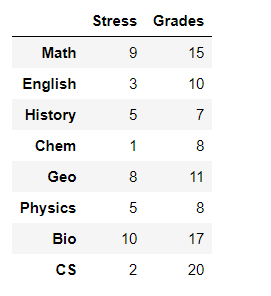Create a line plot that shows the relationships between these three variables.

Code:

## Python3

 `# use plot() method on the dataframe. ``# No parameters are passed so it uses``# variables given in the dataframe``df.plot()`

Output: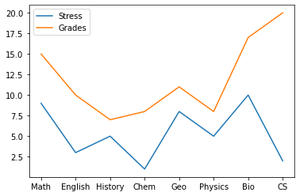An alternative way would be to use gca() method from matplotlib.pyplot library as follows:

## Python3

 `import` `pandas as pd``import` `matplotlib.pyplot as plt`` ` `# Create a list of data``# to be represented in x-axis``subjects ``=` `[ ``'Math'` `, ``'English'` `, ``'History '``,``            ``'Chem'` `, ``'Geo'` `, ``'Physics'` `, ``'Bio'` `, ``'CS'` `]`` ` `# Create a list of data``# to be represented in y-axis``stress ``=` `[ ``9``, ``3` `, ``5` `, ``1` `, ``8` `, ``5` `, ``10` `, ``2` `]`` ` `# Create second list of data to be represented in y-axis``grades ``=` `[ ``15``, ``10` `, ``7` `, ``8` `, ``11` `, ``8` `, ``17` `, ``20` `]`` ` `# Create a dataframe using the two lists``df_days_calories ``=` `pd.DataFrame(``    ``{ ``'Subject'` `: subjects , ``     ``'Stress'``: stress , ``     ``'Grade'``: grades})`` ` `ax ``=` `plt.gca()`` ` `#use plot() method on the dataframe``df_days_calories.plot( x ``=` `'Subject'` `, y ``=` `'Stress'``, ax ``=` `ax )``df_days_calories.plot( x ``=` `'Subject'` `, y ``=` `'Grade'` `, ax ``=` `ax )`

Output:Example 3:

In this example, we will create a plot without explicitly defining variable lists. We will also add a title and change the color.
A coin collector initially has 30 coins. After that, for a duration of one month, he finds one coin every day. Show in a line plot how many coins he has each day of that month.

## Python3

 `import` `pandas as pd`` ` `#initialize the temperature value at the first day of the month``c ``=` `30`` ` `# Create a dataframe using the three lists``# the y-axis variable is a list created using``# a for loops, in each iteration, ``# it adds 1 to previous value``# the x-axis variable is a list of values ranging``# from 1 to 31 (31 not included) with a step of 1``df ``=` `pd.DataFrame([ c ``+` `x ``for` `x ``in` `range``( ``0` `, ``30` `)],``                  ``index ``=` `[``*``range``( ``1` `, ``31` `, ``1` `)],``                  ``columns ``=` `[ ``'Temperature (C)'` `])`` ` `# use plot() method on the dataframe. ``# No parameters are passed so it uses ``# variables given in the dataframe``df.plot(color``=``'red'``, title ``=` `'Total Coins per Day'``)`

Output: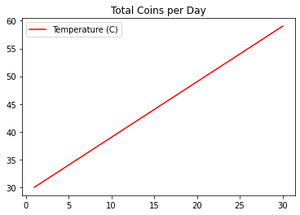Example 4:

In this example, we will plot specific columns of a dataframe. The dataframe consists of three lists, however, we will select two lists only to add to the plot.

Code:

## Python3

 `import` `pandas as pd`` ` `# Create a dataframe using three lists``df ``=` `pd.DataFrame(``    ``{``'List1'``: [ ``1` `, ``2` `, ``3` `, ``4` `, ``5` `, ``6` `], ``     ``'List2'``: [ ``5` `, ``10` `, ``15` `, ``20` `, ``25` `, ``30` `], ``     ``'List3'``: [ ``'a'` `, ``'b'` `, ``'c'` `, ``'d'` `, ``'e'` `, ``'f'` `]})`` ` `# use plot() method on the dataframe.``# List3 is in the x-axis and List2 in the y-axis``df.plot( ``'List3'` `, ``'List2'` `)`

Output: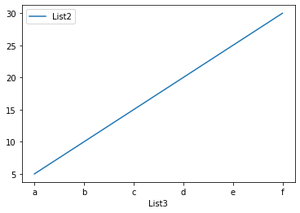Attention geek! Strengthen your foundations with the Python Programming Foundation Course and learn the basics.

To begin with, your interview preparations Enhance your Data Structures concepts with the Python DS Course.

My Personal Notes arrow_drop_up